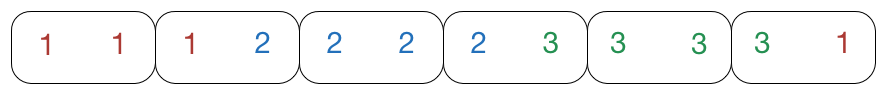# On the Domino Train

Probability Level 5Consider a set of $\frac{ N (N+1) } { 2 }$ dominos which have faces of values 1 to N.
A train of dominos is a straight line of them, in which any 2 touching dominos display the same value.
Let $F(N)$ be the minimum numbers of trains that are needed to use up all of these dominos. What is the value of $F(2016)$?

As an explicit example, $F(3) = 1$ because we have the train listed in the above image.

×